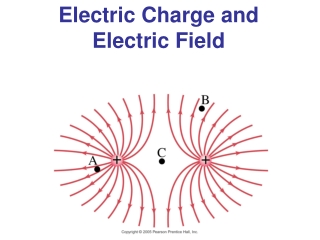DownloadDownload PresentationElectric Charge and Electric Field

# Electric Charge and Electric Field

Download Presentation## Electric Charge and Electric Field

- - - - - - - - - - - - - - - - - - - - - - - - - - - E N D - - - - - - - - - - - - - - - - - - - - - - - - - - -
##### Presentation Transcript

1. Electric Charge and Electric Field

2. Static Electricity; Electric Charge and Its Conservation • Electric Charge in the Atom • Insulators and Conductors • Induced Charge; the Electroscope • Coulomb’s Law • Solving Problems Involving Coulomb’s Law and Vectors • The Electric Field

3. Field Lines • Electric Fields and Conductors

4. Static Electricity; Electric Charge and Its Conservation Objects can be charged by rubbing

5. Static Electricity; Electric Charge and Its Conservation Charge comes in two types, positive and negative; like charges repel and opposite charges attract

6. Static Electricity; Electric Charge and Its Conservation Electric charge is conserved – the arithmetic sum of the total charge cannot change in any interaction.

7. Electric Charge in the Atom Atom: Nucleus (small, massive, positive charge) Electron cloud (large, very low density, negative charge)

8. Electric Charge in the Atom Atom is electrically neutral. Rubbing charges objects by moving electrons from one to the other.

9. Electric Charge in the Atom Polar molecule: neutral overall, but charge not evenly distributed

10. Insulators and Conductors Conductor: Charge flows freely Metals Insulator: Almost no charge flows Most other materials Some materials are semiconductors.

11. Induced Charge; the Electroscope Metal objects can be charged by conduction:

12. Induced Charge; the Electroscope They can also be charged by induction:

13. Induced Charge; the Electroscope Nonconductors won’t become charged by conduction or induction, but will experience charge separation:

14. Induced Charge; the Electroscope The electroscope can be used for detecting charge:

15. Induced Charge; the Electroscope The electroscope can be charged either by conduction or by induction.

16. Induced Charge; the Electroscope The charged electroscope can then be used to determine the sign of an unknown charge.

17. Coulomb’s Law Experiment shows that the electric force between two charges is proportional to the product of the charges and inversely proportional to the distance between them.

18. Coulomb’s Law Coulomb’s law: This equation gives the magnitude of the force.

19. Coulomb’s Law The force is along the line connecting the charges, and is attractive if the charges are opposite, and repulsive if they are the same.

20. Coulomb’s Law Unit of charge: coulomb, C The proportionality constant in Coulomb’s law is then: Charges produced by rubbing are typically around a microcoulomb:

21. Coulomb’s Law Charge on the electron: Electric charge is quantized in units of the electron charge.

22. Coulomb’s Law The proportionality constant k can also be written in terms of , the permittivity of free space:

23. Coulomb’s Law Coulomb’s law strictly applies only to point charges. Superposition: for multiple point charges, the forces on each charge from every other charge can be calculated and then added as vectors.

24. Solving Problems Involving Coulomb’s Law and Vectors The net force on a charge is the vector sum of all the forces acting on it.

25. Solving Problems Involving Coulomb’s Law and Vectors Vector addition review:

26. The Electric Field The electric field is the force on a small charge, divided by the charge:

27. The Electric Field For a point charge:

28. The Electric Field Force on a point charge in an electric field: Superposition principle for electric fields:

29. The Electric Field Problem solving in electrostatics: electric forces and electric fields • Draw a diagram; show all charges, with signs, and electric fields and forces with directions • Calculate forces using Coulomb’s law • Add forces vectorially to get result

30. Field Lines The electric field can be represented by field lines. These lines start on a positive charge and end on a negative charge.

31. Field Lines The number of field lines starting (ending) on a positive (negative) charge is proportional to the magnitude of the charge. The electric field is stronger where the field lines are closer together.

32. Field Lines Electric dipole: two equal charges, opposite in sign:

33. Field Lines The electric field between two closely spaced, oppositely charged parallel plates is constant.

34. Field Lines Summary of field lines: • Field lines indicate the direction of the field; the field is tangent to the line. • The magnitude of the field is proportional to the density of the lines. • Field lines start on positive charges and end on negative charges; the number is proportional to the magnitude of the charge.

35. Electric Fields and Conductors The static electric field inside a conductor is zero – if it were not, the charges would move. The net charge on a conductor is on its surface.

36. Electric Fields and Conductors The electric field is perpendicular to the surface of a conductor – again, if it were not, charges would move.

37. Summary • Two kinds of electric charge – positive and negative • Charge is conserved • Charge on electron: • Conductors: electrons free to move • Insulators: nonconductors

38. Summary • Charge is quantized in units of e • Objects can be charged by conduction or induction • Coulomb’s law: • Electric field is force per unit charge:

39. Summary • Electric field of a point charge: • Electric field can be represented by electric field lines • Static electric field inside conductor is zero; surface field is perpendicular to surface